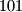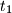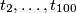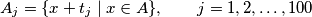IMO Shortlist 2003 problem C1

Kvaliteta:
Avg: 3,0
Težina:
Avg: 6,0
Let$A$ be a$101$-element subset of the set$S=\{1,2,\ldots,1000000\}$. Prove that there exist numbers$t_1$,$t_2, \ldots, t_{100}$ in$S$ such that the setsare pairwise disjoint.
Izvor: Međunarodna matematička olimpijada, shortlist 2003